# OECD Meat Consumption Data Analysis 8 - Serial Correlation Robust Inference using RPhoto by T o T on Unsplash

This post is following of above post.

In this post, I will check if there is serial correlation in the previous regression model.

First, I make residual with resid() function.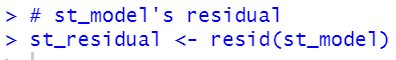Then, let's make a graph for the residual.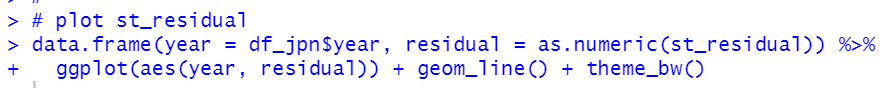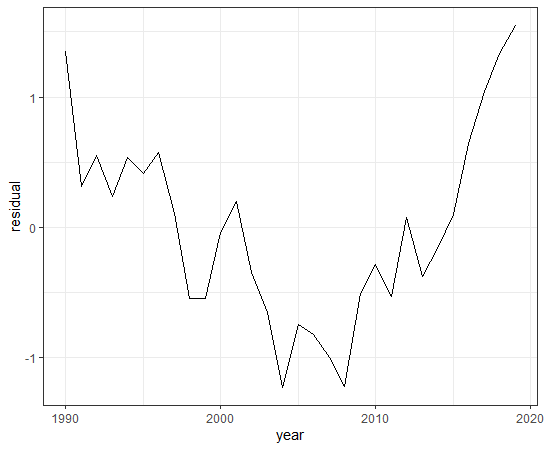I cannot tell if there is serial correlation by watching above graph.

Let's check it.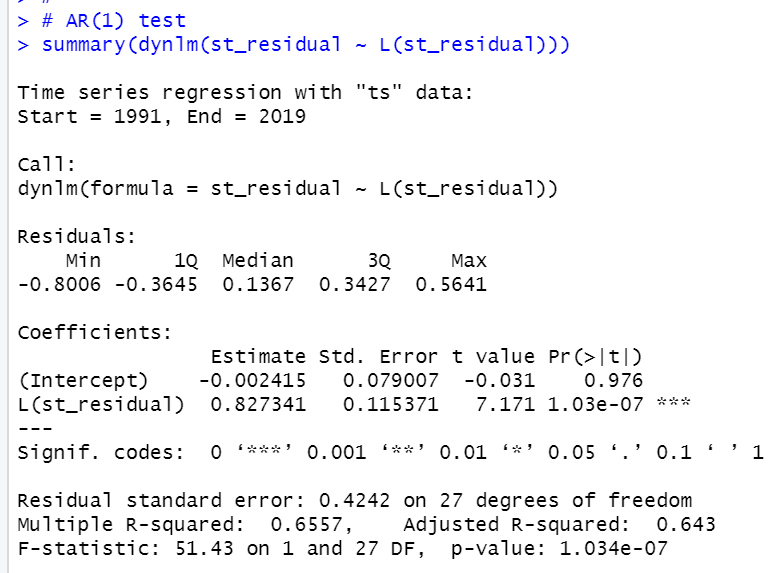st_residual and L(st_residual) are highly correlated. So, st_model has serial correlation.

So, let's make Serial Correlation Robust Inference with lmtest package and sandwich package.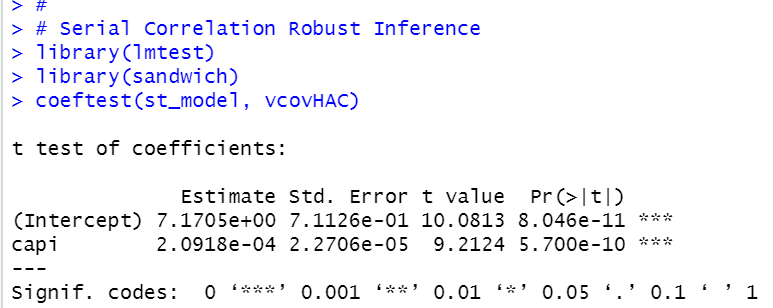capi is still statistically significant, t-value is 9.2124.

Let's see usual inference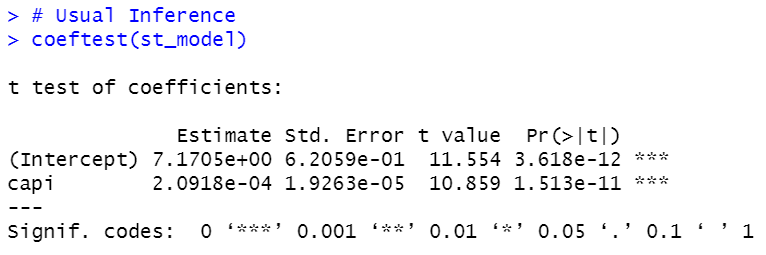capi's t-value is 10.859, it is greater than serial correlation robust inference.

That's it. Thank you!